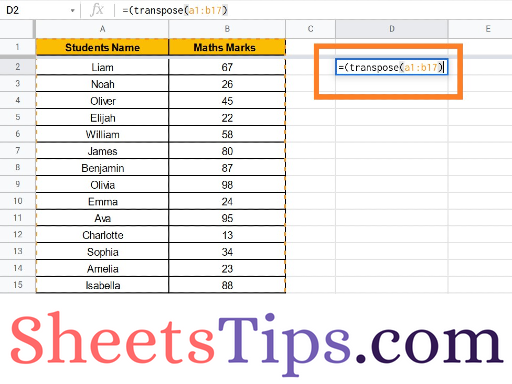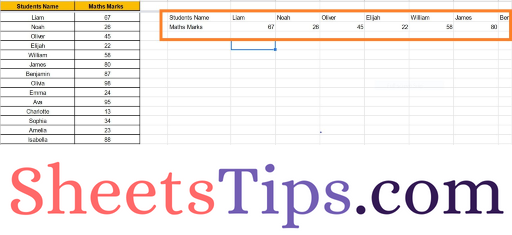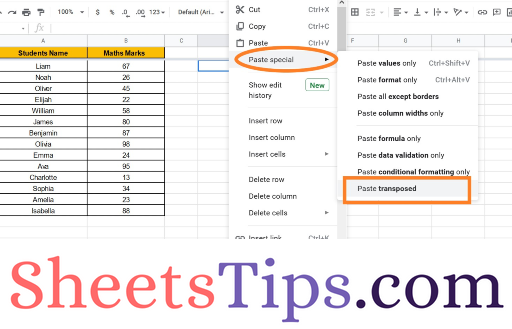# How to Convert Rows to Columns or Backwards in Google Sheets?

When a user is dealing with a large number of Spreadsheets, it is quite common for one to convert rows to columns or backward. Google Sheets allows users to convert rows to columns and column to rows using Google Transpose Function. As the name suggests, the Transpose Function in Google Sheets causes (two or more things) to exchange their places in a spreadsheet.

Let us discuss everything about how to turn columns into rows and rows into columns using the Google Sheets Tips provided on this page. Read further to find more.

## How to Use Google Sheets Transpose Formula?

It is quite simple to use the Transpose Formula in Google Sheets. All we need to do is affix the equal to (=) symbol before the term TRANSPOSE and simply mention the row or the column number which needs to be transposed or exchanged.

Transpose Syntax: =TRANSPOSE(Row/Column)

### How to Convert Rows to Columns in Google Sheets?

• Step 1: Open the Google Spreadsheet where you want to turn rows and columns.
• Step 2: Usually most will already have the data and just need to convert them. However, if your dataset doesn’t have any data, then simply add some data to use the Transpose function.
• Step 3: Now move to the place where you want to convert rows and columns.
• Step 4: Enter the formula “=TRANSPOSE(Cell No)”.
• Step 5: Press the Return Key and now will see the results.In the previous section, we discussed how to convert rows and columns in Google Sheets. Now, what if you want to convert multiple rows and columns at one go. Well, we can achieve the same using the Google Sheets TRANSPOSE function.

Consider the following dataset where we have multiple columns and we need to convert them back to rows. The steps to get this done are as follows:

• Step 1: Open the Google Sheets.
• Step 2: Now move to the cell where you want to transpose the data.
• Step 3: Enter the formula =TRANSPOSE(Cell Range). In our case, the cell range is A1 to B16.• Step 4: Press the “Enter” button and you will see the results.### Easy Trick To Convert Row To Column without Transpose Function

We can also convert row to column or column to row using the Paste option. Let us understand how to get this done by following the steps given below: